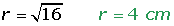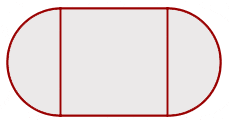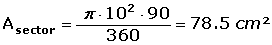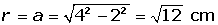## Exercise 1

The wheel of a truck has a radius of 90 cm. How far has the truck travelled when the wheel has rotated 100 times?

## Exercise 2

A lighthouse sweeps its light across the horizon with an angle of rotation of 128 degrees. If the maximum range of the beacon is 7 miles, what is the maximum length of the corresponding arc?

## Exercise 3

A circle has a circumference of 43.96 cm. What is the area of the circle?

## Exercise 4

The area of a circular sector of 90° is 4π cm. Find the radius and length of the circle to which this sector belongs to.

## Exercise 5

Calculate the area of a circular sector whose chord is the side of an inscribed equilateral triangle in a circle with a 2 cm radius.

## Exercise 6

The surface of a table consists of a square of 1 m per side and two semicircles attached on either opposite end. Calculate the area of the table.

## Exercise 7

Calculate the shaded area of the figure below if the radius of the larger circle is 6 cm and the radius of the smaller circles are 2 cm.## Exercise 8

Find the area of the shaded portion of the square ABCD if AB = 10 cm, and APC, AQC are center circle arcs from their centre points B and D respectively.## Exercise 9

Calculate the area of the square inscribed in a circle with a circumference of 18.84 cm.

## Exercise 10

A regular hexagon of side 4 cm has a circle inscribed and another circumscribed around its shape. Find the area enclosed between these two concentric circles.

## Solution of exercise 1

The wheel of a truck has a radius of 90 cm. How far has the truck travelled when the wheel has rotated 100 times?

r = 90 : 100 = 0.9 m

L = 2 · π · 0.9 = 5.65 m

5.65 · 100 = 565 m

## Solution of exercise 2

A lighthouse sweeps its light across the horizon with an angle of rotation of 128 degrees. If the maximum range of the beacon is 7 miles, what is the maximum length of the corresponding arc?## Solution of exercise 3

A circle has a circumference of 43.96 cm. What is the area of the circle?## Solution of exercise 4

The area of a circular sector of 90° is 4π cm. Find the radius and length of the circle to which this sector belongs to.## Solution of exercise 5

Calculate the area of a circular sector whose chord is the side of an inscribed equilateral triangle in a circle with a 2 cm radius.## Solution of exercise 6

The surface of a table consists of a square of 1 m per side and two semicircles attached on either opposite end. Calculate the area of the table.## Solution of exercise 7

Calculate the shaded area of the figure below if the radius of the larger circle is 6 cm and the radius of the smaller circles are 2 cm.## Solution of exercise 8

Find the area of the shaded portion of the square ABCD if AB = 10 cm, and APC, AQC are center circle arcs from their centre points B and D respectively.The shaded portion consists of two circular segments.Calculate the area of both circular segments if the circular area of the segment = area of the circular sector − area of triangle.## Solution of exercise 9

Calculate the area of the square inscribed in a circle with a circumference of 18.84 cm.## Solution of exercise 10

A regular hexagon of side 4 cm has a circle inscribed and another circumscribed around its shape. Find the area enclosed between these two concentric circles.Did you like the article?(1 votes, average: 5.00 out of 5)Loading...

Emma

I am passionate about travelling and currently live and work in Paris. I like to spend my time reading, gardening, running, learning languages and exploring new places.

Did you like
this resource?

Bravo!